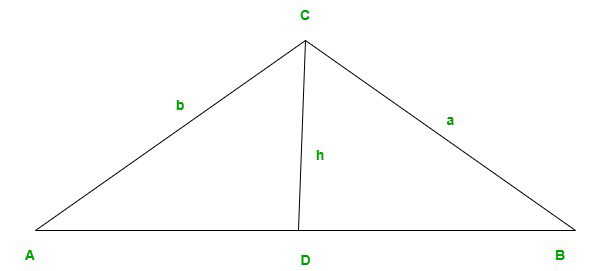What is the Law of Sines?

• Last Updated : 07 Dec, 2021

Geometry is a branch of mathematics that is concerned with the study of shapes, sizes, their parameters, measurement, properties, and relation between points and lines. For two-dimensional shapes represented on a plane, there are three types of geometry. They are Euclidean geometry, Spherical geometry, and Hyperbolic geometry.

The given article discusses the sub-topics of trigonometry. The content of the article includes an explanation of trigonometric ratios, a tabular representation of trigonometric ratios for different angles, an explanation of the Laws of Sine along with its mathematical derivation, and some sample problems for better understanding.

Trigonometry and trigonometric ratios

Trigonometry is a branch of mathematics that deals with the evaluation of sides and angles of a right-angled triangle. Trigonometric operations are conducted with respect to the sides and angles of the given right-angled triangle and some standard trigonometric ratios.

Trigonometric ratios are the numerical values of trigonometric functions based on the ratio of sides in a right-angled triangle. The three sides of the right-angled triangle on which these ratios depend are hypotenuse, perpendicular, and base. The six trigonometric ratios are

• Sine (sin)
• Cosine (cos)
• Tangent (tan)
• Cotangent (cot)
• Cosecant (cosec)
• Secant (sec)

Table of trigonometric ratios

In trigonometric operations, some specific values are commonly used for angle ‘θ’. The trigonometric ratio table includes the value of trigonometric ratios with angle 0°, 30°,45°, 60°, and 90°.

What is the law of sines?

The Law of sines is also widely known as sine law, sine rule, and sine formula. The Law of sines is defined as the ratio of sides of a triangle and their equivalent or opposite sine angles. It is used to determine the unknown side or range of an oblique triangle. For the calculation using the Law of Sines at least, two angles are needed,The other formula derived with respect to the law of sine is,

• a:b:c = sinA : sinB : sinC
• a/b = sinA/sinB
• b/c = sinB/sinC

Derivation of law of sines

Here, a right-angled triangle is required to prove the given trigonometric function.LetABC be a triangle with sides AB = c, BC = a, and AC = b.

Now, draw a perpendicular, CD perpendicular to AB. Then, CD = h which is the height of the triangle.

The perpendicular CD divided \triangleABC into two right-angled triangles CDA and CDB.

To demonstrate: a/b = sinA/sinB

In theCDA,

sinA = h/b

And, in triangle CDB,

sinB = h/a

Therefore,

sinA/sinB = (h/b) / (h/a)

sinA/sinB = a/b

Hence,proved.

Similarly, sinB/sinC = b/c canbe proved and any other pair of angles with respect to the opposite sides.

Sample Problems

Question 1: What do you mean by sine of an angle?

Sine of a angle is defined as a ratio of perpendicular side which stands opposite to thew angle of hypotenuse.

Question 2: Why is the law of sines used?

Law of sines is used to determine the unknown angle or side of a triangle when other two angles and sides are given.

Question 3: What is the cosine rule if a,b and c are the sides and A, B, and C are the angles of the triangle?

If a, b and c are the sides and A, B and C are the angles of a triangle, then cosine rule is given by:

• a2 = b2 + c2 – 2bc cos A
• b2 = a2 + c2 – 2ac cos B
• c2 = a2 + b2 – 2ab cos C

Question 4: What are the three basic trigonometric functions?